PLASMA KEY PARAMETERS

No.
Parameter
Method
1

Plasma resistivity, N series only

The plasma resistivity RB (ohmic part of the plasma bulk resistance) is: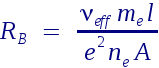with: ne: electron density

neff: effective electron collision rate

me: electron mass

l: effective length of plasma (approx. electron gap)

A: electrode area

NEED
2

Electron collision rate, C series only

The collision rate of electrons ne (please see Figure 2.4) depends on:

• Power and pressure (recipe)

• Gas mixture (recipe)

• Impact of electrons on chemistry

• Feedback from chemistry via cross sections and relative concentration of species

and can be calculated for magnetic field B = 0: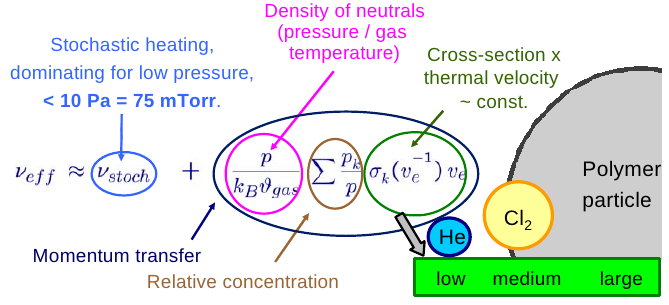The electron collision rate is sensitve to many influences.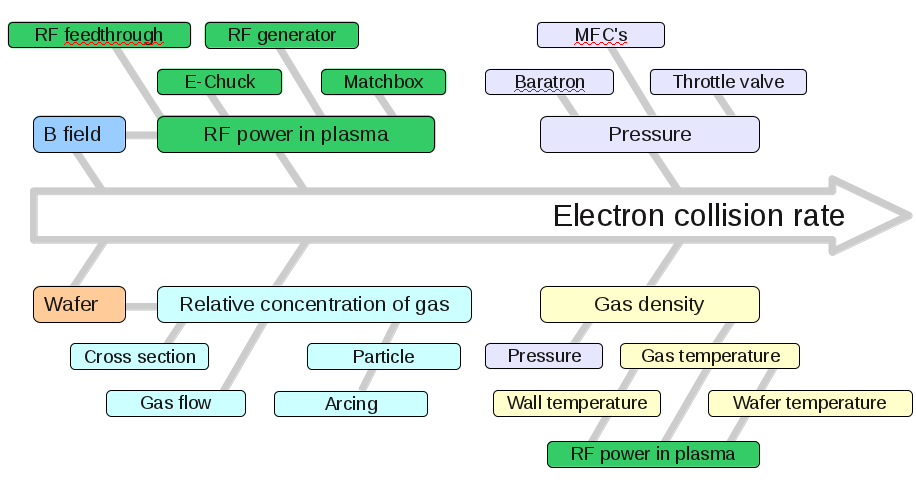SEERS
3

Electron density, C series only

For magnetically enhanced plasmas, the scaling law of electron density is given by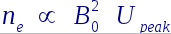Please see M. A. Lieberman, A.J. Lichtenberg, Principles of plasma discharges and materials processing, John Wiley & Sons, Inc., 2005.

Note: The plasma parameters electron density and electron collision rate
only can be calculated by using a broad band measurement because
the inertia effects of the electrons occur only at higher frequencies
(higher harmonics). A separation of these parameters from the plasma
resistivity at lower frequencies as used by the Hercules® N (narrow
band measurement) is not possible.

SEERS
 5

Asymmetry, optional for C series only

The asymmetry of the plasma discharge is defined as the ratio of sheath voltage at the chamber wall to the sheath voltage at the wafer.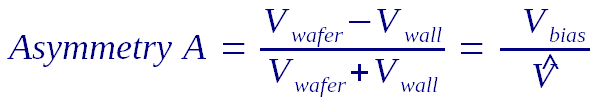Equivalent to DC Bias, not any longer available due to usage of ESC.

A change of asymmetry means always moving charge carriers inside the chamber

- The faster the asymmetry changes, the larger the short time DC current.

- Change of asymmetry --> DC current --> Charging --> Breakdown --> Particle

Larger asymmetry means larger ion energy at the RF driven

SEERS

6

SheathHeating of electrons, optional for C series only

is the net energy gain of electrond per RF cycle through maximum energy of electrons.

Heating or cooling (net gain or loss) of all electrons during one RF cycle in plasma body, close to boundary sheath, given by the energy flux density, defined as:Extraction of the electron heating in the sheath by a plasma physical  model.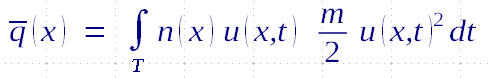provide the so called SheathHeating.

SEERS
7

Resonance Frequency

The resonance frequency is the serial resonance of the bulk 'inductance' and the sheath capacitance and is a property of the plasma. The lower the difference between generator and resonance frequency the higher the dissipated RF power in the plasma bulk. A changed generator frequency influences the resonance conditions of the serial oscillator!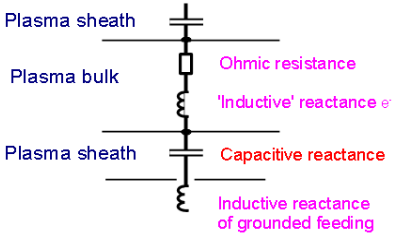If the resonance frequency is more then six times larger than the generator frequency the resonance frequency is setting to the six times larger value.

NEED

8

Uniformity Edge

The parameter Uniformity edge is described by the ratio of the geometric dimensions of the chamber and the skin depth and is frequency depended.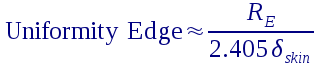NEED
9

Nonlinearity

The parameter nonlinearity describes the ability of the plasma to generate harmonics. The value is usually in the range from 0.05 to 0.25. The nonlinearity is used the calculate the resonance frequency and vice versa. Regularly the nonlinearity is set to 1/8.

NEED
10

RF Fundamentals

The RF Fundamental is the RF current at generator frequency. This parameter indicates variation of the hardware or the RF sub-circuit (electrode system, matchbox, ...).

NEED
11

V1RMS_mV

This parameter shows the amplitude of the sensor signal and is required in order ot checkr functionality of sensor.

NEED